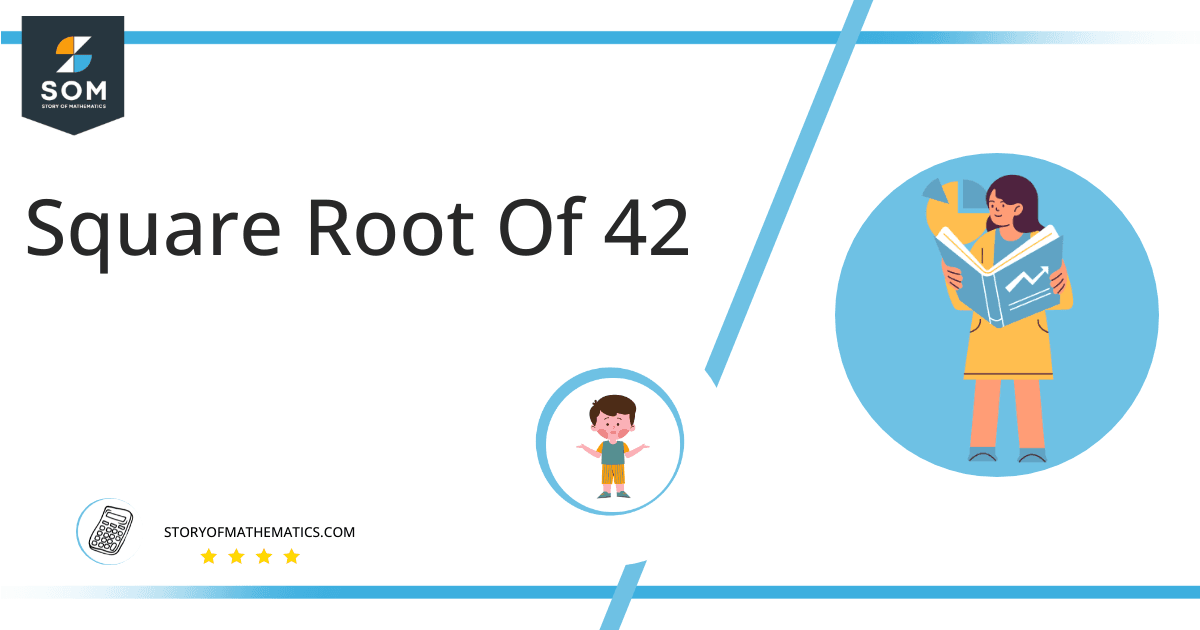# Square Root of 42 + Solution With Free StepsThe square root of 42 is mathematically written as √42 and is equal to 6.48. The sign is known as the square root. The product of two numbers results in the actual number whose square root is being taken. For example, 5 x 5 = 25 which is the required number.

In this article, we will analyze and find the square root of 42 using various mathematical techniques, such as the approximation method and the long division method.

## What Is the Square Root Of 42?

The square root of the number 42 is 6.48.

The square root can be defined as the quantity that can be doubled to produce the square of that similar quantity. In simple words, it can be explained as:

√42 = √(6.48 x 6.48)

√42 = √(6.48)$^2$

√42 = ±6.48

The square can be canceled with the square root as it is equivalent to 1/2; therefore, obtaining 6.48. Hence y is 42’s square root. The square root generates both positive and negative integers.

## How To Calculate the Square Root of 42?

You can calculate the square root of 42 using any of two vastly used techniques in mathematics; one is the Approximation technique, and the other is the Long Division method.

The symbol √ is interpreted as 42 raised to the power 1/2. So any number, when multiplied by itself, produces its square, and when the square root of any squared number is taken, it produces the actual number.

Let us discuss each of them to understand the concepts better.

### Square Root by Long Division Method

The process of long division is one of the most common methods used to find the square roots of a given number. It is easy to comprehend and provides more reliable and accurate answers. The long division method reduces a multi-digit number to its equal parts.

Learning how to find the square root of a number is easy with the long division method. All you need are five primary operations- divide, multiply, subtract, bring down or raise, then repeat.

Following are the simple steps that must be followed to find the square root of 42 using the long division method:

### Step 1

First, write the given number 42 in the division symbol, as shown in figure 1.

### Step 2

Starting from the right side of the number, make pairs of the number 42 such as 42.

### Step 3

Now divide the digit 42 by a number, giving a number either 42 or less than 42. Therefore, in this case, the remainder is 6, whereas the quotient is 6. If the number is not a perfect square, add pair of zeros to the right of the number before starting division.

### Step 4

After this, bring down the next pair 00. Now the dividend is 600. To find the next divisor, we need to double our quotient obtained before. Doubling 6 gives 12; hence consider it as the next divisor.

### Step 5

Now pair 12 with another number to make a new divisor that results in $\leq$ 600 when multiplied with the divisor.

### Step 6

Adding 4 to the divisor and multiplying 124 with 4 results in 496 $\leq$ 600. The remainder obtained is 104. Move the next pair of zeros down and repeat the same process mentioned above.

### Step 7

Keep on repeating the same steps till the zero remainder is obtained or if the division process continues infinitely, solve to two decimal places.

### Step 8

The resulting quotient of 6.48 is the square root of 42. Figure 1 given below shows the long division process in detail:### Square Root by Approximation Method

The approximation method involves guessing the square root of the non-perfect square number by dividing it by the perfect square lesser or greater than that number and taking the average.

The given detailed steps must be followed to find the square root of 42 using the approximation technique.

### Step 1

Consider a perfect square number 36 less than 42.

### Step 2

Now divide 42 by √36.

42 ÷ 6 = 7

### Step 3

Now take the average of 6 and 7. The resulting number is approximately equivalent to the square root of 42.

(6 + 7) ÷ 2 = 6.5### Important points

• The number 42 is not a perfect square.
• The number 42 is an irrational number.
• The number 42 can be split into its prime factorization.

## Is Square Root of 42 a Perfect Square?

The number 42 is not a perfect square. A number is a perfect square if it splits into two equal parts or identical whole numbers. If a number is a perfect square, it is also rational.

A number expressed in p/q form is called a rational number. All the natural numbers are rational. A square root of a perfect square is a whole number; therefore, a perfect square is a rational number.

A number that is not a perfect square is irrational as it is a decimal number. As far as 42 is concerned, it is not a perfect square. It can be proved as below:

Factorization of 42 results in 6 x 7.

Taking the square root of the above expression gives:

= √(6 x 7)

= (6 x 7)$^{1/2}$

= 6.48

This shows that 42 is not a perfect square as it has decimal places; hence it is an irrational number.

Therefore the above discussion proves that the square root of 42 is equivalent to 6.48.Images/mathematical drawings are created with GeoGebra.